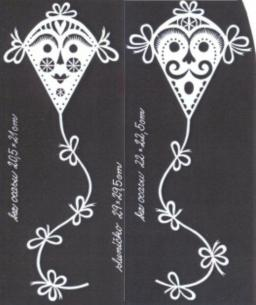# Kite

John a kite, which is diamond shaped. Its diagonals are 60 cm long and 90 cm long. Calculate:

a) the diamond side
b) how much paper John needs to make a kite if he needs paper on both sides and needs 5% of the paper for bending.

Correct result:

a =  54.0833 cm
b =  5670 cm2

#### Solution:We would be pleased if you find an error in the word problem, spelling mistakes, or inaccuracies and send it to us. Thank you!Tips to related online calculators
Pythagorean theorem is the base for the right triangle calculator.

#### You need to know the following knowledge to solve this word math problem:

We encourage you to watch this tutorial video on this math problem:

## Next similar math problems:

• Rhombus and diagonalsThe lengths of the diamond diagonals are e = 48cm, f = 20cm. Calculate the length of its sides.
• RhombusCalculate the perimeter and area of ​​a rhombus whose diagonals are 39 cm and 51 cm long.
• Diagonals of the rhombusHow long are the diagonals e, f in the diamond, if its side is 5 cm long and its area is 20 cm2?
• Diamond sideThe diagonals of the diamond are 18 cm and 14 cm long. Calculate the length of the diamond side.
• Diamond diagonalsCalculate the diamonds' diagonals lengths if the diamond area is 156 cm square and the side length is 13 cm.
• DiagonalsGiven a rhombus ABCD with a diagonalsl length of 8 cm and 12 cm. Calculate the side length and content of the rhombus.
• Diamond diagonalsFind the diamond diagonal's lengths if the area is 156 cm2 and side is 13 cm long.
• Cardboard boxWe want to make a cardboard box shaped quadrangular prism with rhombic base. Rhombus has a side of 5 cm and 8 cm one diagonal long. The height of the box to be 12 cm. The box will be open at the top. How many square centimeters cardboard we need, if we ca
• DiagonalsA diagonal of a rhombus is 20 cm long. If it's one side is 26 cm find the length of the other diagonal.
• Rhombus IVCalculate the length of the diagonals of the rhombus, whose lengths are in the ratio 1: 2 and a rhombus side is 35 cm.
• Total areaCalculate the total area (surface and bases) of a prism whose base is a rhombus which diagonals of 12cm and 18cm and prism height is 10 cm.
• Roof coverAbove the pavilion with a square ground plan with a side length of a = 12 m is a pyramid-shaped roof with a height v = 4.5 m. Calculate how much m2 of sheet metal is needed to cover this roof if 5.5% of the sheet we must add for joints and waste.
• Diamond diagonalsCalculate the diamond's diagonal lengths if its content is 156 cm2 and the side length is 13 cm.
• Rhombus and inscribed circleIt is given a rhombus with side a = 6 cm and the radius of the inscribed circle r = 2 cm. Calculate the length of its two diagonals.
• Rhombus ABCDRhombus ABCD, |AC| = 90 cm, |BD| = 49 cm. Calculate the perimeter of the rhombus ABCD.
• DiamondRhombus has side 17 cm and and one of diagonal 22 cm long. Calculate its area.
• Hexagon areaThe center of the regular hexagon is 21 cm away from its side. Calculate the hexagon side and its area.